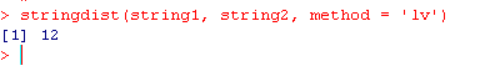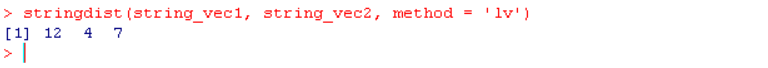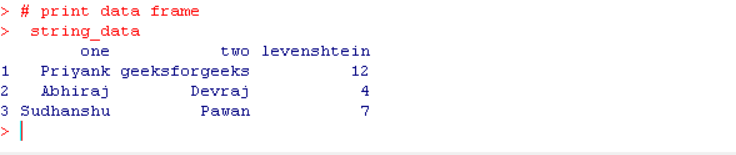Open in App
Not now

# How to Calculate Levenshtein Distance in R?

• Last Updated : 26 Jan, 2022

In this article, we will discuss how to calculate Levenshtein Distance in the R Programming Language.

The Levenshtein distance between two strings is the minimum number of character substitutions, insertions, and deletions required to turn one string into the other string. The Levenshtein distance practically is used in approximate string matching, spell-checking, natural language processing, etc.

To calculate the Levenshtein distance in the R Language, we use the stringdist() function of the stringdist package library. The stringdist package is an R Language library that contains approximate String Matching, Fuzzy Text Search, and String Distance functions. The stringdist() function computes pairwise string distances between two or more strings, vectors, or data frame columns.

## Levenshtein distance between two strings

To calculate Levenshtein distance in the R Language, we use the stringdist() function of the stringdist package library. The stringdist() function takes two strings as arguments and returns the Levenshtein distance between them.

Syntax: stringdist( string1, string2, method=”lv” )

Parameter:

• string1 and string2: determine the string whose Levenshtein distance is to be calculated.

Example: Here, we will calculate the Levenshtein distance between two strings.

## R

 `# load library stringdist ` `library``(stringdist) ` ` `  `# sample strings ` `string1= ``"Priyank"` `string2= ``"geeksforgeeks"` ` `  `# calculate Levenshtein Distance ` `stringdist``(string1, string2, method = ``'lv'``)`

Output:## Levenshtein distance between two string vectors:

To calculate the Levenshtein distance between two vectors in the R Language, we use the stringdist() function of the stringdist package library. The stringdist() function takes two string vectors as arguments and returns a vector that contains the Levenshtein distance between each string pair in them.

Syntax: stringdist( string_vec1, string_vec2, method=”lv” )

Parameter:

• string_vec1 and string_vec2: determine the string vectors whose Levenshtein distance is to be calculated.

Example: Here, we will calculate the Levenshtein distance between two string vectors.

## R

 `# load library stringdist ` `library``(stringdist) ` ` `  `# sample strings ` `string_vec1<- ``c``(``"Priyank"``, ``"Abhiraj"``, ``"Sudhanshu"``) ` `string_vec2<- ``c``(``"geeksforgeeks"``, ``"Devraj"``, ``"Pawan"``) ` ` `  `# calculate Levenshtein Distance ` `stringdist``(string_vec1, string_vec2, method = ``'lv'``)`

Output:## Levenshtein distance between two string columns of a dataframe

To calculate Levenshtein distance between two string columns of a data frame in the R Language, we use the stringdist() function of the stringdist package library. The stringdist() function takes two string columns of a data frame as arguments and returns a vector that contains the Levenshtein distance between them.

Syntax: stringdist( string_data\$column1, string_data\$column2, method=”lv” )

Parameters:

• string_data: determines the data frame containing string columns.
• column1 and column2: determine the string columns of data frame whose Levenshtein distance is to be calculated.

Example: Here, we will calculate the Levenshtein distance between two string columns of a data frame.

## R

 `# load library stringdist ` `library``(stringdist) ` ` `  `# sample string data frame ` `string_data<- ``data.frame``(one= ``c``(``"Priyank"``,  ` `                                ``"Abhiraj"``, ``"Sudhanshu"``), ` `                         ``two= ``c``(``"geeksforgeeks"``,  ` `                                ``"Devraj"``, ``"Pawan"``)) ` ` `  `# calculate Levenshtein Distance ` `string_data\$levenshtein<-``stringdist``(string_data\$one,  ` `                                    ``string_data\$two, ` `                                    ``method = ``'lv'``) ` ` `  `# print data frame ` ` ``string_data`

Output:My Personal Notes arrow_drop_up
Related Articles